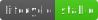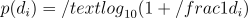# Data auditing: Get started

## Introduction

Welcome to the ‘Data auditing’ vignette of the jfa package. Here you can find a simple explanation of the functions in the package that facilitate data auditing. For more detailed explanations of each function, read the other vignettes on the package website.

## Functions and intended usage

Below you can find an explanation of the available data auditing functions in jfa.

### Test digit distributions with digit_test()The function digit_test() takes a vector of numeric values, extract the requested digits, and compares the frequencies of these digits to a reference distribution. By default, the function performs a frequentist hypothesis test of the null hypothesis that the digits are distributed according to the reference distribution and produces a p value. When a prior is specified, the function performs a Bayesian hypothesis test of the null hypothesis that the digits are distributed according to the reference distribution against the alternative hypothesis that the digits are not distributed according to the reference distribution and produces a Bayes factor (Kass & Raftery, 1995).

Full function with default arguments:

digit_test(x,
check = c("first", "last", "firsttwo"),
reference = "benford",
prior = FALSE)

Supported options for the check argument:

check Returns
fist First digit
firsttwo First and second digit
last Last digit

Supported options for the reference argument:

check Returns
benford Benford’s law
uniform Uniform distribution
Vector of probabilities Custom distribution

Example usage:

Benford’s law (Benford, 1938) is a principle that describes a pattern in many naturally-occurring numbers. According to Benford’s law, each possible leading digit d in a naturally occurring, or non-manipulated, set of numbers occurs with a probability:The distribution of leading digits in a data set of financial transaction values (e.g., the sinoForest data) can be extracted and tested against the expected frequencies under Benford’s law using the code below.

# Frequentist hypothesis test
digit_test(sinoForest$value, check = "first", reference = "benford") ## ## Digit Distribution Test ## ## data: sinoForest$value
## n = 772, MAD = 0.0065981, X-squared = 7.6517, df = 8, p-value = 0.4682
## alternative hypothesis: leading digit(s) are not distributed according to the benford distribution.
# Bayesian hypothesis test using default prior
digit_test(sinoForest$value, check = "first", reference = "benford", prior = TRUE) ## ## Digit Distribution Test ## ## data: sinoForest$value
## n = 772, MAD = 0.0065981, BF10 = 1.4493e-07
## alternative hypothesis: leading digit(s) are not distributed according to the benford distribution.

### Test for repeated values with repeated_test()The function repeated_test() analyzes the frequency with which values get repeated within a set of numbers. Unlike Benford’s law, and its generalizations, this approach examines the entire number at once, not only the first or last digit. For the technical details of this procedure, see Simohnsohn (2019).

Full function with default arguments:

repeated_test(x,
check = "last",
method = "af",
samples = 2000)

Supported options for the check argument:

check Returns
last Last decimal
lasttwo Last two decimals
all All decimals

Supported options for the method argument:

check Returns
af Average frequency
entropy Entropy

Example usage:

In this example, we analyze a data set from a (retracted) paper that describes three experiments run in Chinese factories, where workers were nudged to use more hand-sanitizer. These data were shown to exhibited two classic markers of data tampering: impossibly similar means and the uneven distribution of last digits (Yu, Nelson, & Simohnson, 2018). We can use the rv.test() function to test if these data also contain a greater amount of repeated values than expected if the data were not tampered with.

repeated_test(sanitizer$value, check = "lasttwo", samples = 5000) ## ## Repeated Values Test ## ## data: sanitizer$value
## n = 1600, AF = 1.5225, p-value = 0.0028
## alternative hypothesis: average frequency in data is greater than for random data.

## Benchmarks

To validate the statistical results, jfa’s automated unit tests regularly verify the main output from the package against the following benchmarks: